### Home > A2C > Chapter 2 > Lesson 2.1.4 > Problem2-50

2-50.
1. Solve each equation below. Homework Help ✎

1. 2 − (x + 5) = 7 − 2x + 3

2. (2x + 4)(x − 3) = 0

3.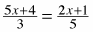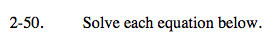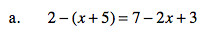Distribute the −1 to (x + 5) and solve.

2 − x − 5 = 7 − 2x + 3

x = 13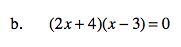Remember the Zero Product Property.

2x + 4 = 0; x − 3 = 0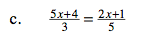Multiply both sides by 15 to eliminate the denominators.

25x + 20 = 6x + 3